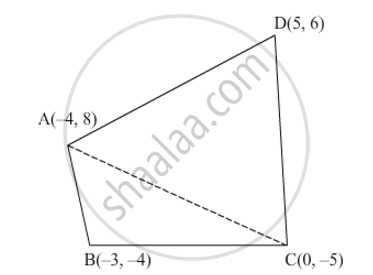Share

# If A(−4, 8), B(−3, −4), C(0, −5) and D(5, 6) are the vertices of a quadrilateral ABCD, find its area. - CBSE Class 10 - Mathematics

#### Question

If A(−4, 8), B(−3, −4), C(0, −5) and D(5, 6) are the vertices of a quadrilateral ABCD, find its area.

#### SolutionLet the vertices of the quadrilateral be A(−4, 8), B(−3, −4), C(0, −5) and D(5, 6).

Join AC to form two triangles, namely, ΔABC and ΔACD.

Area of quadrilateral ABCD = Area of ΔABC + Area of ΔACD

We know

Area of triangle having vertices (x1, y1), (x2, y2) and (x3, y3) =1/2[x1(y2y3)+x2(y3y1)+x3(y1y2)]

Now,

Area of ABC=1/2{(4)[(4)(5)]+(3)[(5)(8)]+(0)[(8)(4)]}

=1/2(4+39)

=35/2

Area of ACD=1/2{(4)[(5)(6)]+(0)[(6)(8)]+(5)[(8)(5)]}

=1/2(44+65)

=109/2

Area of quadrilateral ABCD=Area of ABC+Area of ACD

35/2+109/2

=72 square units

Thus, the area of quadrilateral ABCD is 72 square units.

Is there an error in this question or solution?

#### Video TutorialsVIEW ALL 

Solution If A(−4, 8), B(−3, −4), C(0, −5) and D(5, 6) are the vertices of a quadrilateral ABCD, find its area. Concept: Area of a Triangle.
S